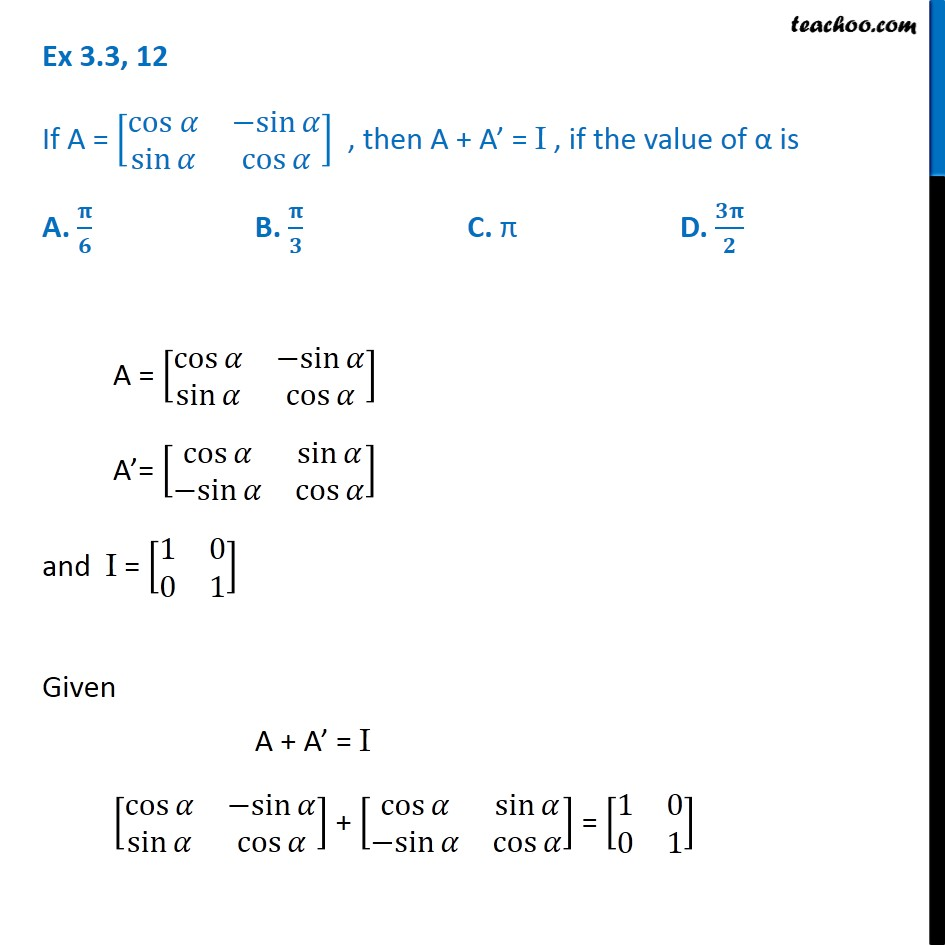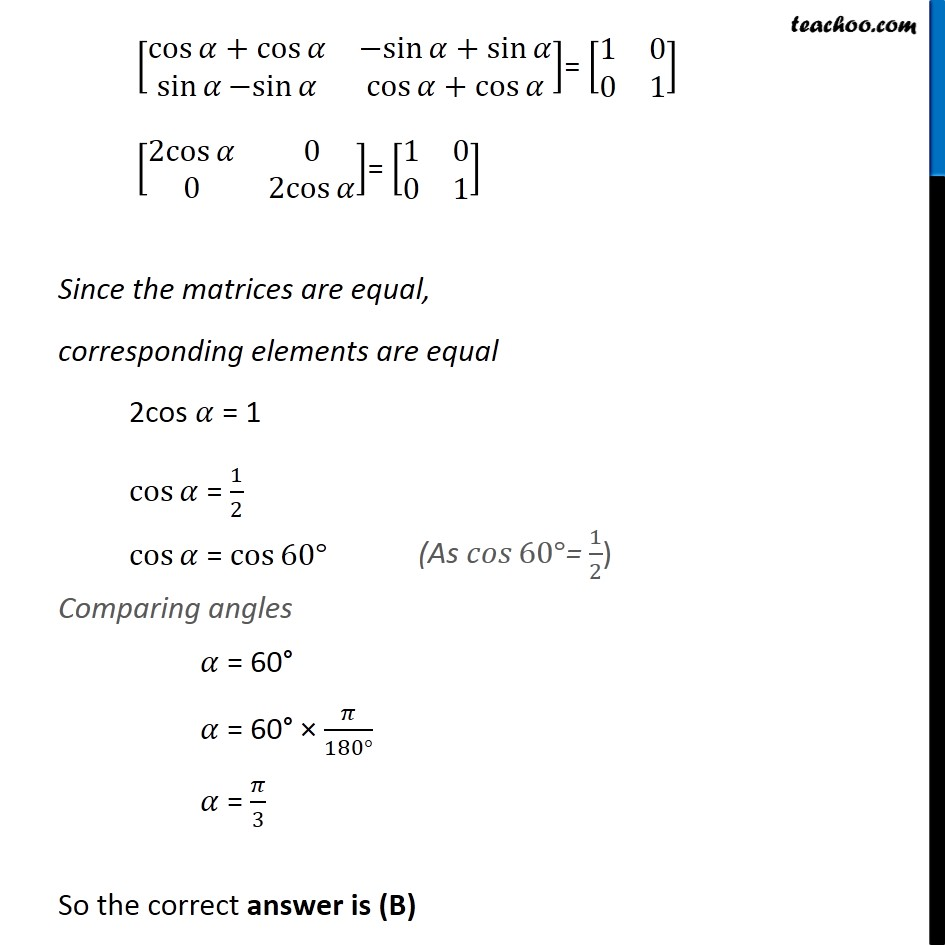1. Class 12
2. Important Questions for exams Class 12
3. Chapter 3 Class 12 Matrices

Transcript

Ex 3.3, 12 If A = [■8(cos 𝛼&〖−sin〗⁡𝛼@sin⁡𝛼&cos⁡𝛼 )] , then A + A’ = I , if the value of α is A. 𝛑/𝟔 B. 𝛑/𝟑 C. π D. 𝟑𝛑/𝟐 A = [■8(cos⁡𝛼&〖−sin〗⁡𝛼@sin⁡𝛼&cos⁡𝛼 )] A’= [■8(cos⁡𝛼&sin⁡𝛼@〖−sin〗⁡𝛼&cos⁡𝛼 )] and I = [■8(1&0@0&1)] Given A + A’ = I [■8(cos⁡𝛼&〖−sin〗⁡𝛼@sin⁡𝛼&cos⁡𝛼 )] + [■8(cos⁡𝛼&sin⁡𝛼@〖−sin〗⁡𝛼&cos⁡𝛼 )] = [■8(1&0@0&1)] [■8(cos⁡〖𝛼+cos⁡𝛼 〗&〖−sin〗⁡〖𝛼+sin⁡𝛼 〗@sin⁡𝛼 〖−sin〗⁡𝛼&cos⁡〖𝛼+cos⁡𝛼 〗 )]= [■8(1&0@0&1)] [■8(2cos⁡𝛼&0@0&2cos⁡𝛼 )]= [■8(1&0@0&1)] Since the matrices are equal, corresponding elements are equal 2cos 𝛼 = 1 cos⁡𝛼 = 1/2 cos⁡𝛼 = cos⁡〖60°〗 Comparing angles 𝛼 = 60° 𝛼 = 60° × 𝜋/(180°) 𝛼 = 𝜋/3 So the correct answer is (B) (As 𝑐𝑜𝑠⁡〖60°〗= 1/2)

Chapter 3 Class 12 Matrices

Class 12
Important Questions for exams Class 12